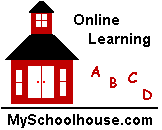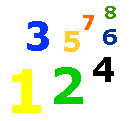# 1-47 The Fact Families 1 to 10

### Lesson List

A fact family is a group of related math facts.

Addition and subtraction are related fact families.

3 + 4 = 7 AND 4 + 3 = 7

7 - 3 = 4 AND 7 - 4 = 3

These four facts are a fact family.

2 + 3 = 5 AND 3 + 2 = 5

5 - 2 = 3 AND 5 - 3 = 2

These four facts are a fact family

Fill in the boxes.  Find the related fact family.

 1 + 0 = 1 + 1 = 11 + 1 = 2 - 1 = 12 + 1 = 3 + 2 = 33 - 1 = 2 3 - 2 =4 - 3 = 1 4 - = 33 + 1 = 4 1 + = 42 + 3 = 5 + 2 = 55 - 3 = 2 - 2 = 34 + 2 = 6 + 4 = 66 - 4 = 2 6 - 2 =5 + 2 = 7 + 5 = 77 - 2 = 5 - 5 = 23 + 5 = 8 + 3 = 88 - 3 = 5 8 - = 37 + 2 = 9 + 7 = 99 - 7 = 2 9 - = 76 + 4 = 10 + 6 = 1010 - 4 = 6 10 - = 4

 Elementary & Middle School Lessons & Self-Correcting Tests for Children in all Subject Areas. If you have found an error or would like to make comments on this lesson, please email us at: MySchoolhouse.online@gmail.com Copyright 1999-2022 by Educational Designers, LLC.  All rights reserved. Lessons & Tests in Math, Reading, Spelling, Science, Language, and Social Studies. "This site uses cookies from Google to deliver its services and analyze traffic. Your IP address and user-agent are shared with Google along with performance and security metrics to ensure quality of service, generate usage statistics, and to detect and address abuse." WORK SPACE